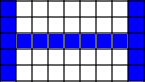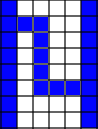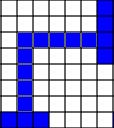# Procedural Dungeon Generation

This is an interactive article, play with the settings. Click on the canvas to reanimate.

Procedural generation has always interested me. From the landscapes of Minecraft to the hundreds of rougelikes, procedural generation makes any game a more unpredictable and brings near infinite replay value to the game. The basic principle is to use a source of randomness - or pseudo-randomness; to create environments according to some set of rules.

## Outline

So, what makes a dungeon? Very simply, rooms connected by corridors. Let’s start with the rooms.

One approach to generate the basic skeleton is to create a number of randomly sized rectangles at some point and then iteratively separate them.

Another way is to use Binary Space Partitioning. We recursively split the available space - either vertically or horizontally; until we reach an ideal size or exceed some depth. Now a room can be a randomly sized rectangle contained within any partition. This relatively simple method that yields pretty decent results. And we don’t have to worry about separating the overlapping rooms since partitions are mutually exclusive.

## Partitions

Each partition can be thought of as a node in a tree that that encloses all its children or a leaf in the tree that is simply a rectangle. A parent partition can be split to make child partitions.

Since splitting evenly will simply give us a uniform set of partitions, we vary the split point by some distance about the middle. When we split parent, we need to pick the direction and ratio. The ratio can be controlled by using the `varf` parameter that is range around midpoint that you want to split in.

``````let f = random.nextFloat(0.5 - varf, 0.5 + varf);
this.children = this.rect.split(f, axis).map(half => {
return new Partition(half, { sqf, varf }, depth - 1);
});
``````

The direction is another way to control the shape of the resultant partitions. Notice that the ratio of `width` to `height` of a square is closer to 1 than a rectangle. To use this to our advantage, we can apply a bias to our `choice()` that is the ratio of the width to the height.

``````let bias = this.rect.w / this.rect.h;
``````

We make a choice to split either vertically or horizontally based on the `bias` and an exponent (`exp`).

``````function choice(bias = 1, exp = 1) {
return Math.random() * Math.pow(bias, exp) < 0.5;
}
``````

Modulate the squariness (`sqf`) to prefer vertical splits when `bias > 1` which gives rise to squarer children.

``````let axis = choice(bias, sqf) ? 'y' : 'x';
``````

## Rooms

From these partitions we can create our rooms. Let’s start with clipping partitions to a grid.

``````for (let part of root) {
part.rect.scale(1 / gridSize).round();
}
``````

Now, we iterate through the leaf nodes of the tree. We first discard any leaves that are too small to make a decent sized room. Then, for each leaf, we take its bounds and make a randomly sized (`w` and `h`) room with random offsets (`x` and `y`). It’s also a good idea to maintain some prescribed minimum spacing (`roomSpace`) between adjacent rooms.

``````let w = random.nextInt(minDim, Math.min(r.w - roomSpace, maxDim));
let h = random.nextInt(minDim, Math.min(r.h - roomSpace, maxDim));
let x = r.x + random.nextInt(roomSpace, r.w - w);
let y = r.y + random.nextInt(roomSpace, r.h - h);
rooms.push(new Room(new Rect(x, y, w, h)));
``````

We can control the bounds on the dimensions of the rooms we make using the `minDim` and `maxDim` parameters.

We could also modify the recursive split function to further split spaces that are larger than what we want and discard smaller spaces.

## Spans

Now that we have our rooms, we have to connect them. A simple minimum spanning tree will suffice for this.

Initialize sets to keep track of `remaining` and `connected` rooms. Starting with the first room in `connected` and the rest in `remaining`.

``````let connected = new Set(rooms.slice(0, 1));
let remaining = new Set(rooms.slice(1));
``````

Then, keep connecting the nearest unconnected room until there are none left.

``````while (remaining.size != 0) {
let [room, nearest] = findNearest(connected, remaining);
let pair = new Set([room, nearest]);
if (!corridors.has(pair)) {
this.corridors.set(pair, true);
remaining.delete(nearest);
connected.add(nearest);
}
}
``````

## Corridors

For each span, we also have to plot a corridor between the two rooms.

If there is an overlap (on any axis) between the rooms, then we have two sides facing each other which we can connect in two different ways - either straight or staggered. We can make a random choice between either of these with a bias (`straightBias`) parameter to control their relative distribution.Straight CorridorStaggered Corridor

But, if there is no overlap, then we fallback to connecting the rooms with a right-angled corridor.Right-Angled Corridor

And that’s pretty much it, you now have the blueprint of a dungeon. Check out the full source code.